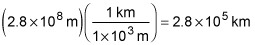##### Physics I: Practice Problems For DummiesUsing physics, you can calculate the orbital speed and radius of an object as it revolves around another one. For example, given the orbital speed of a satellite around Earth, you can calculate the satellite's orbital radius.

Here are some practice questions that you can try.

## Practice questions

1. A satellite orbits Earth at an altitude of 400 kilometers above the planet's surface. What is its speed in meters per second?

2. The GMVX satellite is "pulled" along by the force of Earth's gravity at a speed of 1,200 meters per second. How many kilometers from Earth's center is the GMVX located?

The following are the answers to the practice questions:

1.Use the orbital velocity equation: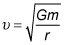where G is the gravitational constant, m is the mass of the central body — in this case, Earth — and r is the distance from the satellite to Earth's center (in meters). First, convert 400 kilometers to the correct units: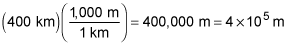If the radius of Earth is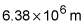then the distance r from the center of Earth to the satellite is: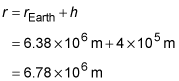Here, h is the altitude of the satellite above the Earth's surface.

Therefore, substituting that value along with the mass of Earth into the orbital velocity equation yields: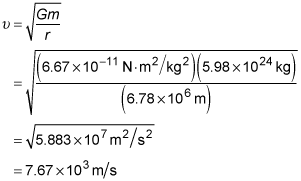2.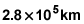Use the orbital velocity equation,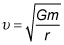where m is the mass of the orbited body (here, Earth) and r is the distance the orbiting object (the satellite) is separated from the center of the body it's orbiting.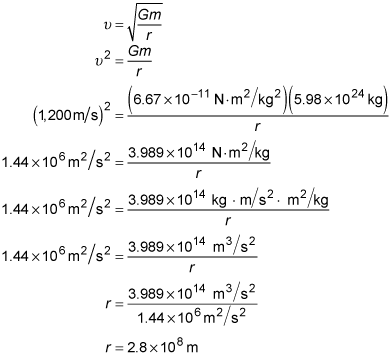Now convert to kilometers: